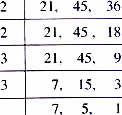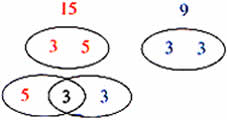Tuesday , July 5 2022# NCERT 5th Class (CBSE) Mathematics: Factors And Multiples

### Using LCM

1. Find the smallest number which when divided by 21, 45 and 36 will leave a remainder of 7 in each case.

We need to find the least common multiple of these numbers and then 7 to it so that there is a remainder of 7 when we divide by these numbers.If we divide 1260 by 21, 45 and 36 we will not get a reminder. In order to have reminder of 7, we need to add 7 to the LCM. That is 1260+7 = 1267

Answer: The smallest number when divided by 21, 36 and 45 that will leave a reminder of 7 is 1267.

## RELATIONSHIP BETWEEN HCF AND LCM

Find the HCF and LCM of 15 and 9.

15 = 3×5
9 = 3×3HCF = 3and  LCM = 3×3×5 = 45

Multiply the HCF and LCM

45×3 = 135

Multiply the two numbers

15×9 = 135

The product of two numbers is equal to the product of their HCF and LCM.

You can use this relationship to find the numbers when you know their HCF and LCM.

### Example

Two numbers have their HCF as 4 and their LCM as 48. If one of the numbers is 16, find the other number.

HCF×LCM = Given number × the other number

HCF × LCM = the product of the numbers

4×48 = 192

16×? = 192

Divide to find out.

192÷16 = 12

Answer: The other number is 12.

## चुनौती हिमालय की 5th NCERT CBSE Hindi Rimjhim Ch 18

चुनौती हिमालय की 5th Class NCERT CBSE Hindi Book Rimjhim Chapter 18 प्रश्न: लद्दाख जम्मू-कश्मीर राज्य में …